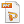Contact

Back to Presentations

# Error in the likelihood ratio: false match probability

M.W. Perlin, "Error in the likelihood ratio: false match probability", American Academy of Forensic Sciences 69th Annual Meeting, New Orleans, LA, 17-Feb-2017.Download HandoutDownload PowerPoint

Abstract

After attending this presentation, attendees will understand why false match probability is important in forensic science, and how the FMP can be accurately and rapidly calculated.

This presentation will impact the forensic science and criminal justice communities by showing how to quantify in error in forensic analysis and DNA mixture interpretation.

In identification science, there can be a very many possible types. Observables that exist in the physical world can be conceptualized as random samples drawn from a type space. Probability and information statements made about observed types refer to the full sample space of all possible types.

A prior probability distribution describes the chance of observing a type before examining data. A posterior distribution over type space updates these probabilities based on examined data. The Bayes factor of a type in the sample space is a ratio of posterior to prior probabilities.

When stating a factor for a type exemplar relative to evidence, there is a chance of false match error. With a DNA mixture, this error is the probability of misidentifying a non-contributor type as a contributor, when the type coincidentally has a factor value at least as large as a given match statistic.

The probability of falsely matching a wrong exemplar by chance is the false match probability (FMP). The error can be quantified by calculating the subset size of misidentified types having spuriously large factors.

The FMP can be costly to calculate exactly on a large type space. However, when a type is formed from a independent subtypes, the factor is a numerical product of the subtype factor values. Independence helps with rapid and accurate calculation of the joint factor distribution. Evaluating the joint distribution’s tail probability beyond a fixed factor value measures the type subset showing false matches.

A trier of fact does not want to make a mistake by wrongly convicting an innocent person. Most jurors do not know Bayes theorem or statistical independence. Few have studied mathematical probability, and fewer still have learned conditional probability. They rarely know about likelihood (the probability of data given a hypothesis), much less the likelihood ratio (LR) factor that contrasts two competing hypotheses. But they do understand the chance of error, and they want to avoid making a mistake.

Considering all the people in the world, what is the chance that a reported match statistic identifies the wrong person? DNA mathematics can randomly embed the seven and a half billion (1010) people in the world in a dense space of a trillion trillion (1024) possible genotypes. Population genetics can estimate prior genotype probability, while Bayesian update on evidence data can produce a posterior genotype distribution. Prior and posterior combine to give a factor function over all genotype space.

The factor function's inverse connects extreme match values to an error subset of types. The one dimensional tail probability of extreme match values is the multidimensional measure of non-contributor types. Actual objects in the physical world have types that are samples from the full type space. To determine a false match probability relative to all the people in the world, it is computationally effective to reduce the function to a single variable and its tail probability.

The LR summarizes the probative value of evidence in forensic identification. The FMP puts an error rate to that LR value, customized to the evidence in a particular case. Both numbers are important to a trier of fact – the LR's strength of match, and the FMP's chance of error. While 1/LR is always an upper bound on LR error, calculating the FMP can provide an exact estimate of misidentification frequency. The FMP gives additional error information about an LR match statistic, simply expressed as the chance of making a mistake.

The author will present the FMP method, and demonstrate its use in analyzing DNA in a sexual assault case and searching a DNA database. Understanding match statistic error will help an analyst better quantify the chance of making a mistake.